Next: k nearest neighbor Up: Vector space classification Previous: Document representations and measures   Contents   Index

# Rocchio classification

Figure 14.1 shows three classes, China, UK and Kenya, in a two-dimensional (2D) space. Documents are shown as circles, diamonds and X's. The boundaries in the figure, which we call decision boundaries , are chosen to separate the three classes, but are otherwise arbitrary. To classify a new document, depicted as a star in the figure, we determine the region it occurs in and assign it the class of that region - China in this case. Our task in vector space classification is to devise algorithms that compute good boundaries where good'' means high classification accuracy on data unseen during training.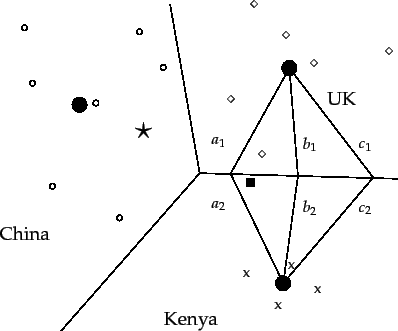Perhaps the best-known way of computing good class boundaries is Rocchio classification , which uses centroids to define the boundaries. The centroid of a class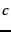is computed as the vector average or center of mass of its members: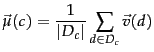(139)

where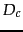is the set of documents in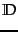whose class is: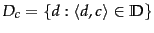. We denote the normalized vector of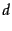by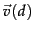(Equation 25 , page 6.3.1 ). Three example centroids are shown as solid circles in Figure 14.3 .

The boundary between two classes in Rocchio classification is the set of points with equal distance from the two centroids. For example,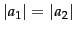,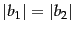, and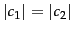in the figure. This set of points is always a line. The generalization of a line in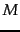-dimensional space is a hyperplane, which we define as the set of points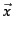that satisfy: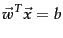(140)

where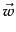is the-dimensional normal vector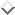of the hyperplane and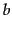is a constant. This definition of hyperplanes includes lines (any line in 2D can be defined by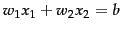) and 2-dimensional planes (any plane in 3D can be defined by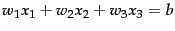). A line divides a plane in two, a plane divides 3-dimensional space in two, and hyperplanes divide higher-dimensional spaces in two.

Thus, the boundaries of class regions in Rocchio classification are hyperplanes. The classification rule in Rocchio is to classify a point in accordance with the region it falls into. Equivalently, we determine the centroid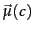that the point is closest to and then assign it to. As an example, consider the star in Figure 14.3 . It is located in the China region of the space and Rocchio therefore assigns it to China. We show the Rocchio algorithm in pseudocode in Figure 14.4 .

Table 14.1: Vectors and class centroids for the data in Table 13.1 .
 term weights vector Chinese Japan Tokyo Macao Beijing Shanghai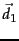0 0 0 0 1.0 0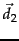0 0 0 0 0 1.0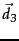0 0 0 1.0 0 0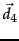0 0.71 0.71 0 0 0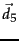0 0.71 0.71 0 0 0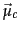0 0 0 0.33 0.33 0.33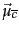0 0.71 0.71 0 0 0

Worked example. Table 14.1 shows the tf-idf vector representations of the five documents in Table 13.1 (page 13.1 ), using the formula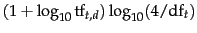if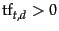(Equation 29, page 6.4.1 ). The two class centroids are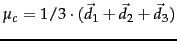and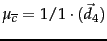. The distances of the test document from the centroids are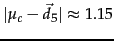and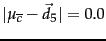. Thus, Rocchio assigns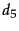to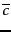.

The separating hyperplane in this case has the following parameters: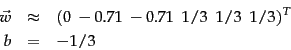See Exercise 14.8 for how to computeand. We can easily verify that this hyperplane separates the documents as desired: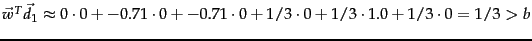(and, similarly,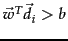for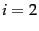and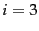) and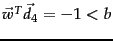. Thus, documents inare above the hyperplane (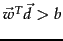) and documents inare below the hyperplane (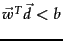).

End worked example.

The assignment criterion in Figure 14.4 is Euclidean distance (APPLYROCCHIO, line 1). An alternative is cosine similarity: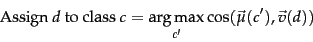(141)

As discussed in Section 14.1 , the two assignment criteria will sometimes make different classification decisions. We present the Euclidean distance variant of Rocchio classification here because it emphasizes Rocchio's close correspondence to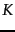-means clustering (Section 16.4 , page 16.4 ).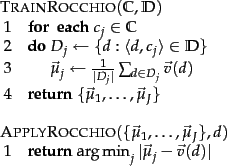Rocchio classification is a form of Rocchio relevance feedback (Section 9.1.1 , page 9.1.1 ). The average of the relevant documents, corresponding to the most important component of the Rocchio vector in relevance feedback (Equation 49, page 49 ), is the centroid of the class'' of relevant documents. We omit the query component of the Rocchio formula in Rocchio classification since there is no query in text classification. Rocchio classification can be applied to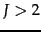classes whereas Rocchio relevance feedback is designed to distinguish only two classes, relevant and nonrelevant.

In addition to respecting contiguity, the classes in Rocchio classification must be approximate spheres with similar radii. In Figure 14.3 , the solid square just below the boundary between UK and Kenya is a better fit for the class UK since UK is more scattered than Kenya. But Rocchio assigns it to Kenya because it ignores details of the distribution of points in a class and only uses distance from the centroid for classification.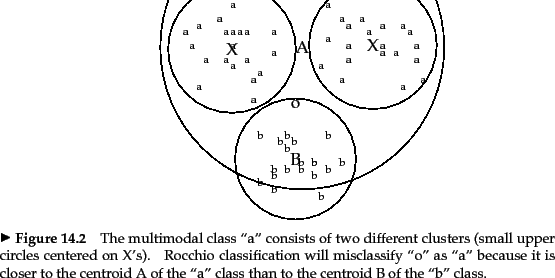The assumption of sphericity also does not hold in Figure 14.5 . We cannot represent the a'' class well with a single prototype because it has two clusters. Rocchio often misclassifies this type of multimodal class . A text classification example for multimodality is a country like Burma, which changed its name to Myanmar in 1989. The two clusters before and after the name change need not be close to each other in space. We also encountered the problem of multimodality in relevance feedback (Section 9.1.2 , page 9.1.3 ).

Two-class classification is another case where classes are rarely distributed like spheres with similar radii. Most two-class classifiers distinguish between a class like China that occupies a small region of the space and its widely scattered complement. Assuming equal radii will result in a large number of false positives. Most two-class classification problems therefore require a modified decision rule of the form: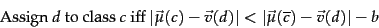(142)

for a positive constant. As in Rocchio relevance feedback, the centroid of the negative documents is often not used at all, so that the decision criterion simplifies to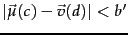for a positive constant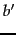.

 mode time complexity training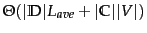testing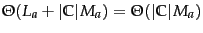Training and test times for Rocchio classification.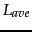is the average number of tokens per document.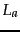and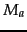are the numbers of tokens and types, respectively, in the test document. Computing Euclidean distance between the class centroids and a document is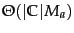.

Table 14.2 gives the time complexity of Rocchio classification.Adding all documents to their respective (unnormalized) centroid is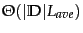(as opposed to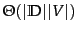) since we need only consider non-zero entries. Dividing each vector sum by the size of its class to compute the centroid is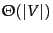. Overall, training time is linear in the size of the collection (cf. Exercise 13.2.1 ). Thus, Rocchio classification and Naive Bayes have the same linear training time complexity.

In the next section, we will introduce another vector space classification method, kNN, that deals better with classes that have non-spherical, disconnected or other irregular shapes.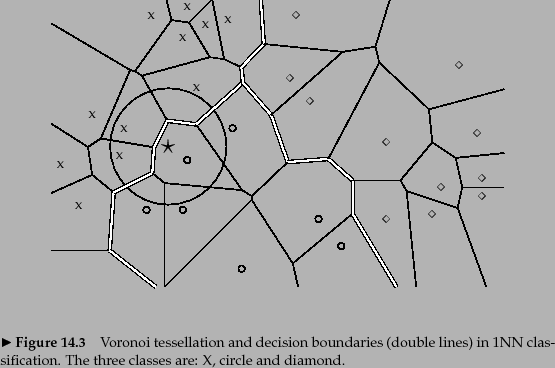Exercises.

• Show that Rocchio classification can assign a label to a document that is different from its training set label.Next: k nearest neighbor Up: Vector space classification Previous: Document representations and measures   Contents   Index